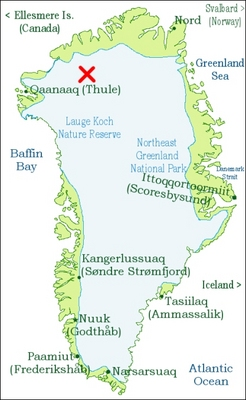A physicist in Australia has come up with an experiment that could potentially reveal a flaw in Newton's law of gravitation. If the flaw exists, it would be the first evidence in support of theories that explain the movement of galaxies without having to introduce "dark matter".

For the past 70 years or so, physicists have been bothered by a nagging question: why do the centres of galaxies rotate too fast for the amount of mass we can see through telescopes? The most popular answer is that most of the mass is hidden in large bands of "dark matter", a substance that is invisible because it doesn't interact strongly with light. If it exists, dark matter could account for 95% of the mass in galaxies, and would explain many other aspects of the universe.

However, a lack of evidence for dark matter has led a small camp of physicists to promote an alternative answer: the gravitational force that holds galaxies together decays more gently with distance than presently estimated, meaning that Newton's law of gravitation is not quite as simple as an inverse-square relationship. The theory, which is known as modified Newtonian dynamics (MOND), proposes adding extra factors to Newton's 300-year-old equations so that the gravitational behaviour only alters at very low accelerations. Unfortunately the turmoil of gravitational forces produced in the galaxy means that such accelerations are hard to come by, leaving proponents of MOND with no easy way to test their theory.©PhysicsWeb Modified Newtonian dynamics (MOND) is a theory that tries to account for the strange rotation of galaxies by assuming that Newton's gravitational law is incorrect at very low accelerations. On Earth it is hard to find situations of truly low acceleration because all the objects in the galaxy are pulling each other with gravity. However, Alex Ignatiev from the Theoretical Physics Research Institute in Melbourne in Australia has discovered that, twice a year, the Solar System happens to reach a certain geometry that cancels-out most of the conflicting forces at two places on the Earth's surface. On the 22 September next year, one of these places will be at 56° west longitude and 79°50' north latitude, in the far north of Greenland (marked above with a red cross).

However, Alex Ignatiev from the Theoretical Physics Research Institute in Melbourne claims to have predicted instances on the Earth where most of these forces will cancel out. Ignatiev first considered how an object at rest in the centre-of-mass of our galaxy would appear to be accelerating when viewed from a laboratory on Earth. This involved listing all the major accelerations such as the Earth's rotation around the Sun and the Sun's orbit in our galaxy. He then looked for solutions where all of the accelerations add up to zero.

The solutions indicated that, on either of the two annual equinoxes, there will be two places on the Earth's surface where the force cancellation occurs. For example, on the equinox of 22 September 2008, one will be in the far north of Greenland and the other will be on the opposite side of the world in Antarctica (see figure: "X marks the spot"). Ignatiev says that if a gravitational wave detector is set-up to monitor a static test object at one of these times and places, it might just be able to glimpse a tiny, 0.2 × 10-16 m deflection over a period of 0.5 ms - what he calls "SHLEM" (static high-latitude equinox modified inertia). If SHLEM is observed, it would be the first evidence in support of MOND.

"Even if the result were negative it would be a very significant step forward, because an interesting theory would be ruled out," Ignatiev told Physics Web. "But if the predicted SHLEM effect were observed - well, we'd have to rewrite our most basic theories."